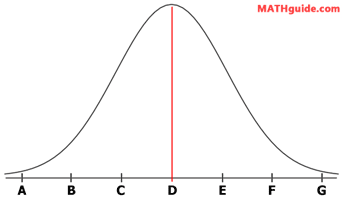Bell Curve: Standard Deviation
View the Lesson | MATHguide homepage Updated March 6th, 2018

Status: Waiting for your answers.

Problem: Given a mean of 80.2 and a standard deviation of 2.3, determine the values marked A - F on the bell curve below that correspond to three standard deviations.Solution:
 A: B: C: D: E: F: G: